library(sharpshootR)
library(daymetr)
library(soilDB)

Background

Cumulative PPT over the course of an idealized water year (October 1 through September 30). Measurements are selected from water years with annual PPT close to the 5th, 25th, 50th, 75th, and 95th percentiles of annual PPT (over all water years). Key elements:

• red line is the current water year’s cumulative PPT trajectory
• red date and value are current date and cumulative PPT
• red box-whisker are 5-25-50-75-95th percentiles of cumulative PPT on current day of water year
• blue traces are cumulative PPT records corresponding to 5th, 25th, 50th, 75th, and 95th percentiles of historical (end of water-year) cumulative PPT
• blue box-whisker indicates 5-25-50-75-95th percentiles of starting water-day where cumulative PPT > 0.1 (units)

TODO: relate to these excellent maps: https://cw3e.ucsd.edu/odds-of-normal-water-year-precipitation/

Various Data Sources

CDEC

# define station of interest
s <- 'SPW'
s.info <- CDEC_StationInfo(s)
# format title for cumulative PPT
title.text <- sprintf("%s [%s]", s.info$site.meta$Name, s)

# get data
x <- CDECquery(id=s, sensor=45, interval='D', start='2000-01-01', end='2030-01-01')

## NOTE: requires sharpshootR >= 1.4.02
# plot
par(mar=c(4.5, 4.5, 2.5, 1.5))
PCP_plot(x, ylab='Cumulative PPT (inches)', main=title.text, this.year = 2020)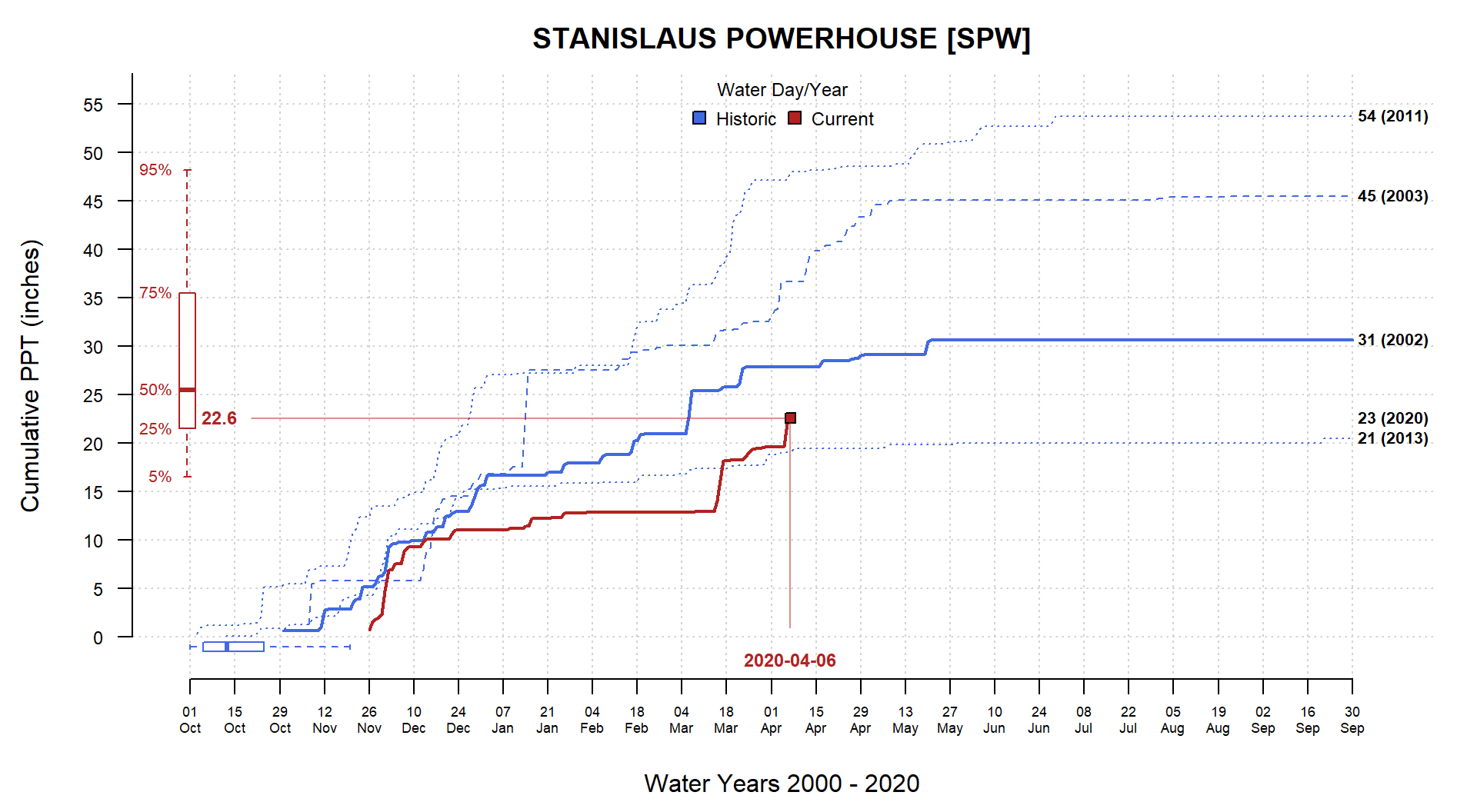DAYMET

Using daily precipitation data near Sonora, CA (-120.3822, 37.9829), from DAYMET point-query API.

# convenience function
getDaymet <- function(x, y, start_yr, end_yr) {

# daymet point query
lat = y,
lon = x,
start = start_yr,
end = end_yr,
internal = TRUE
)

# keep only the data
d <- d$data # format datetime d$datetime <- as.Date(sprintf('%s %s', d$year, d$yday), format="%Y %j")

# extract water year and water day
w <- waterDayYear(d$datetime) # add back to original data, row-order is preserved d$water_year <- w$wy d$water_day <- w$wd # extract PPT and convert to inches d$value <- d$prcp..mm.day. / 25.4 return(d) } # get the data x <- getDaymet(x=-120.3822, y=37.9829, start_yr=1981, end_yr=2017) # examples par(mar=c(4.5, 4.5, 2.5, 1.5)) PCP_plot(x, ylab='Cumulative PPT (in)', main='DAYMET', this.year = 2017)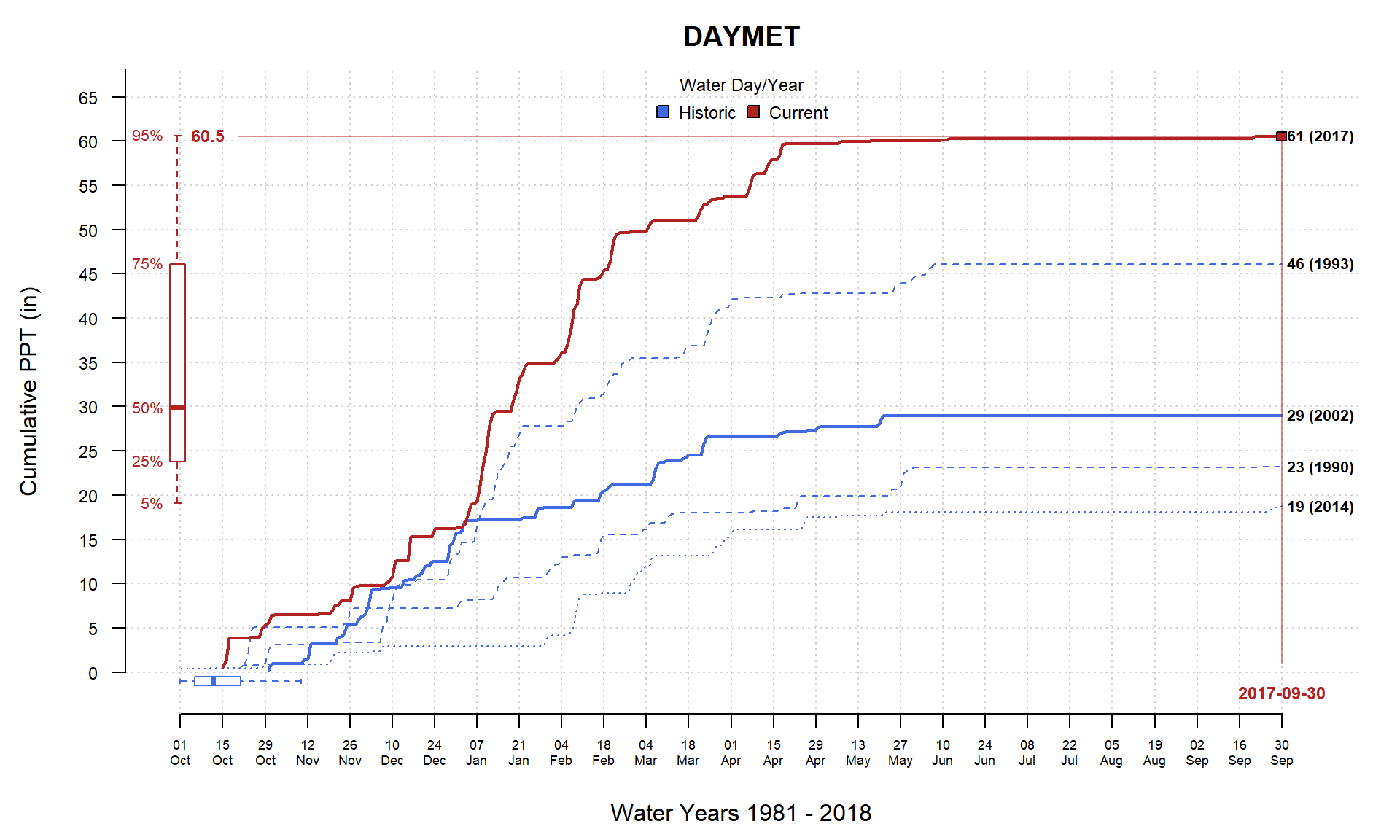PCP_plot(x, ylab='Cumulative PPT (in)', main='DAYMET', this.year = 2017, this.day=50)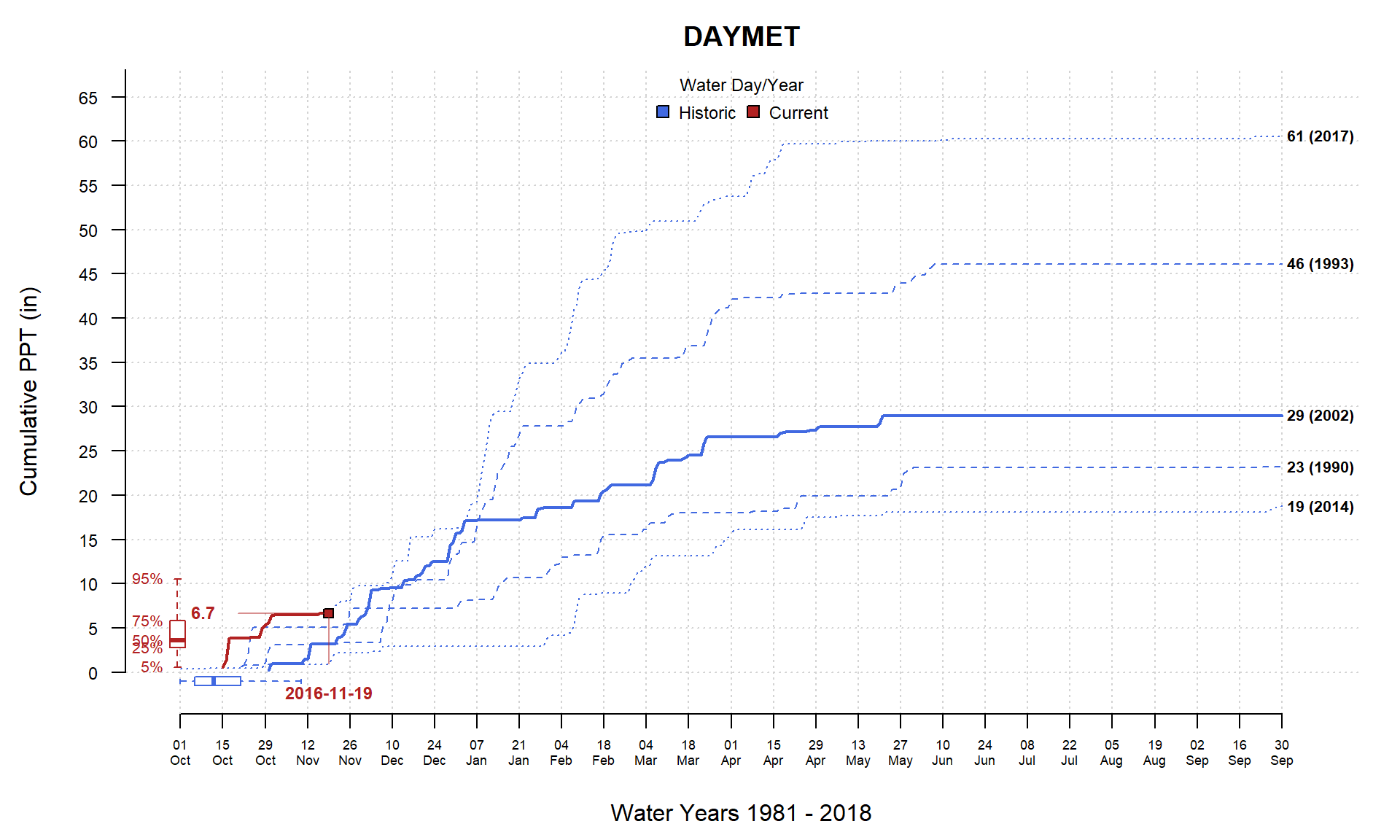PCP_plot(x, ylab='Cumulative PPT (in)', main='DAYMET', this.year = 1999, this.day = 100)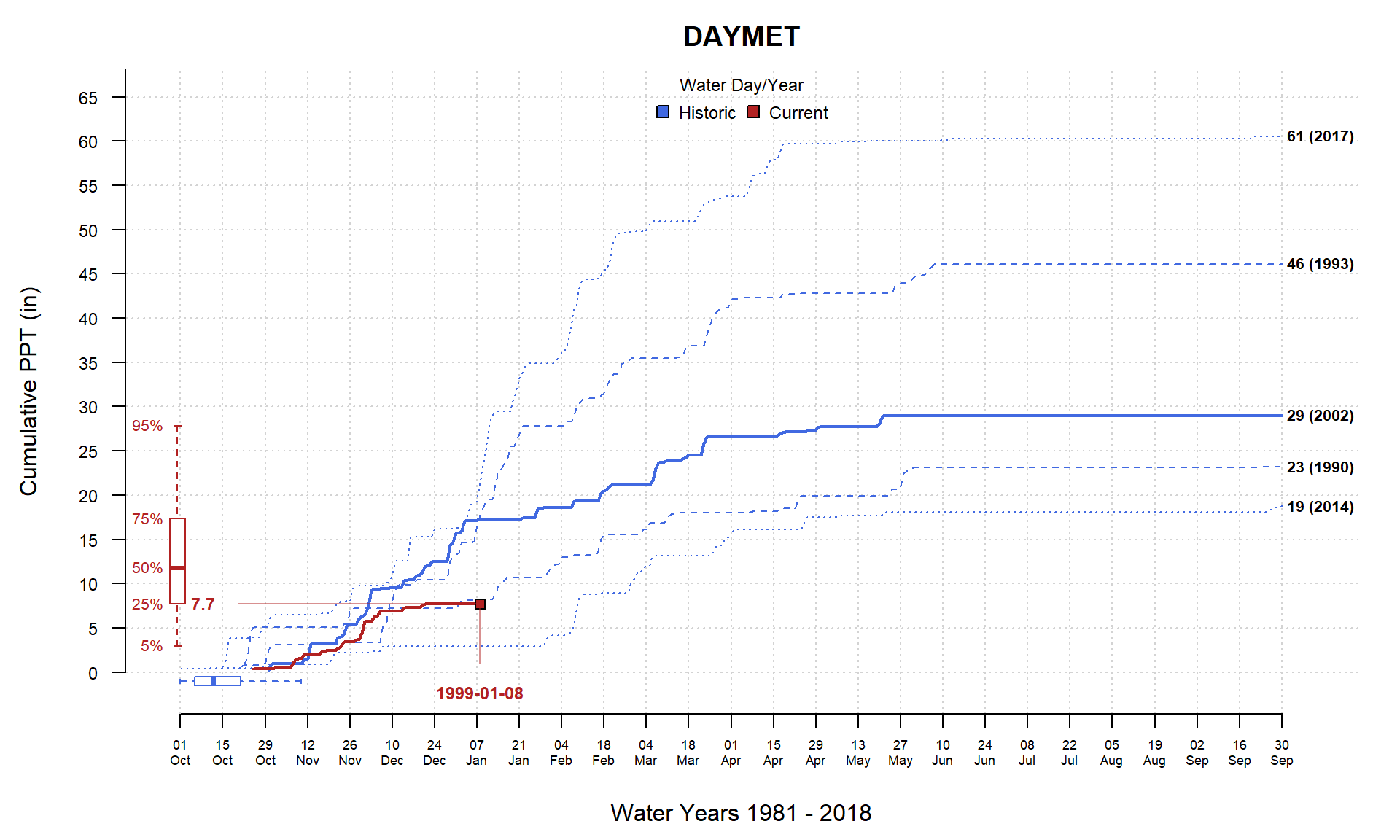SCAN/SNOTEL Daily climate data from the Rogers Farm #1 SCAN station; site number 2001. # range can extend beyond actual records x <- fetchSCAN(site.code=c(2001), year=1990:2020) # extract instantanious precipitation, units are inches x <- x$PRCP
# copy date into column expected by PCP_plot
x$datetime <- x$Date

par(mar=c(4.5, 4.5, 2.5, 1.5))
PCP_plot(x, ylab='Cumulative PPT (in)', main='SCAN Station 2001', this.year = 2019, this.day = 300)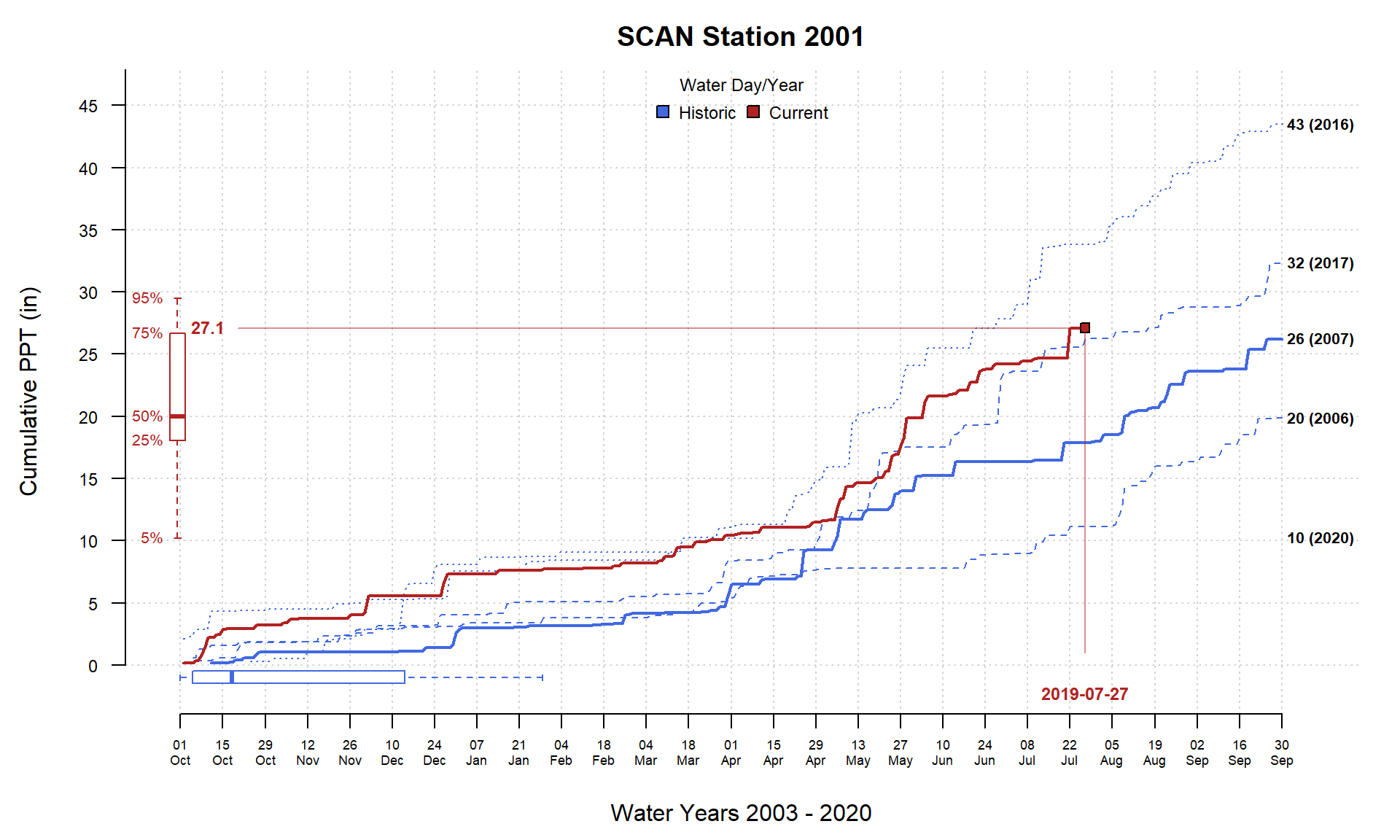Animations

TODO.

This document is based on sharpshootR version 1.6.1.